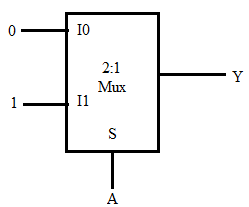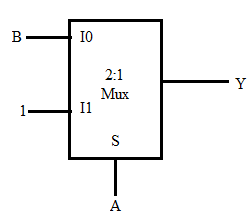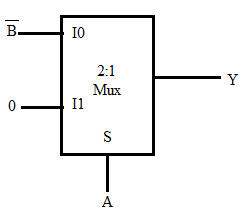## Index

 STA & SI Chapter1 Chapter2 Chapter3 Chapter4 Chapter5 Chapter6 Chapter7 Chapter8 Introduction Static Timing Analysis Clock Advance STA Signal Integrity EDA Tools Timing Models Other Topics

 Extraction & DFM Chapter1 Chapter2 Chapter3 Chapter4 Chapter5 Chapter6 Introduction Parasitic Interconnect Corner (RC Corner) Manufacturing Effects and Their Modeling Dielectric Layer Process Variation Other Topic

## Wednesday, December 25, 2013

### DIGITAL BASIC - 1.5 : Multiplexer (MUX)

 Index Chapter1 Chapter2 Chapter3 Chapter4 Digital Background Semiconductor Background CMOS Processing

 1.1 1.2 1.3a 1.3b 1.4 1.5 1.6 Number System Digital Arithmetic Logic Gates Logic Gates Combinational Circuits Multiplex (MUX)

De-Multiplexer:
• Receives information on a single line and transmits that information on one of 2n possible output lines.
• The selection of specific output line is controlled by the bit values of ‘n’ selection lines.
Multiplexer:
• Multiplexing means transmitting a large number of information units over a smaller number of channels lines.
• A digital multiplexer is a combinational circuit that selects binary information from one of many inputs lines and directs it in a signal output line.
• The selection of a particular line is controlled by a set of selection lines.
• Normally, there are 2n input lines and ‘n’ selection lines whose bit combinations determine which input is selected.”
• Multiplexers can be used for the implementation of Boolean functions, combinational circuits. They can also used for parallel to serial conversion.
• Multiplexer is also called data selector or universal circuit.
• It is used for connection two or more sources to a single destination among computer units and it is useful for constructing a common bus system

Important Points:
To implement 2n :1 MUX by using 2:1 MUX, the total number or 2:1 MUX required is 2n-1

 Given MUX To be implementedMUX RequiredNo of MUX 4 : 1 16 : 1 4+1=5 4 : 1 64 : 1 16+4+1=21 8 : 1 64 : 1 8+1 =9 8 : 1 256 : 1 32+4+1=37

Implementation of Higher Order MUX using Lower Order MUX:
4:1 MUX by 2:1 MUX

Total number of 2: 1 MUX = 3

MUX as a universal logic gate

 Gate Type Implemented by MUX  + Equation Buffer Y=output = ANOT/Inverter Y=A’AND Y=A.BOR Y=A+BNOR Y=(A+B)’NAND Y=(A.B)’XORXNORImplementation of Boolean function using Multiplexer:
The Boolean function may be implemented in 2n to 1 multiplexer.
• If we have a Boolean function of n variables, we take n-1 of these variables and connect them to the selection lines of a multiplexer (let’s say these are “select variables”).
• The remaining single variable (MSB variable) of the function is used for the inputs of the multiplexer (let’s say these are “input variable”).
• Now form the implementation table
• First row lists all those minterms where “input variable” is complemented (say 0).
• Second row lists all those minterms where “input variable” is in its normal form (say 1).
• The minterms are circled as per the given Boolean function. Now use the following steps to find out final multiplexer inputs.
• If the 2 minterms in a column are not circled, 0 is placed to the corresponding multiplexer inputs.
• If the 2 minterms in a column are circled, 1 is placed to the corresponding multiplexer inputs.
• If the minterms in the second row is circled and the first row is not circled, apply second row of variable to the corresponding multiplexer inputs.
• If the minterms in the first row is circled and not the second row, apply first row of the variable to the corresponding multiplexer inputs.
Example:  Implementation of given function using 8 to 1 multiplexer
F(A,B,C,D) = Ʃ (1,3,4,11,12,13,14,15)
Solution.
• Total number of variable n = 4 (A,B,C,D)
• Number of select lines:  n-1= 3 (B, C, D)
• The given function has 4 variable, so 16 possible minterms (0 – 15) are entered in the implementation table.
• All the minterms are divided into 2 groups
• The first group (0-7) minterms  are entered in the first row  (Variable A =0)
• The second group (8–15) minterms are entered in the second row (Variable A= 1)
• Circle the minterm number as per function, which you have to implement (in this case it’s 1,3,4,11,12,13,14,15)
• Find out the multiplexer input as per above given steps.

Implementation Table

Given multiplexer is 8:1
Logic diagram

Example
Implement the following Boolean function using 8 : 1 MUX
F(A,B,C,D) = Ʃ m(0,1,2,4,6,9,12,14)
Solution.
Select lines are B, C and D

Follow all the steps as per above points.

Example
Implement the following Boolean function with 8 : 1 multiplexer
F(A,B,C,D) = ∏M (0,3,5,6,8,9,10,12,14)

Solution
The given maxterms are inverted to obtain minterms. From the minterms, we can implement the above Boolean function by using 8 : 1 multiplexer. Select lines are B, C and D, the input variable is A.
F(A,B,C,D) = Ʃ m(1,2,4,7,11,13,15)

Example
Implement the following Boolean function with 8 : 1 multiplexer
F(A,B,C,D) = Ʃ m (0,2,6,10,11,12,13) + Ʃ d(3,8,14)
Solution.
The Boolean function has three don’t care conditions which can be treated as either 0’s or 1’s. In this example don’t care condition is consider as 1.

1.Very good explaination

2.very helpful..are you going to post remaining concepts of digital designing

1.Yes I will do. but it will take some time.

3.How many 16:1mux required for 64:1 mux

1.[64/16]+[64/16²] = 4

4.Someone gave me answer as 4 -64/16= 4
And some as 5- 64/16=4+1(for next one)=5
So which one is correct 5 or 4

1.if you can't use anyother gate.. like AND, OR, XOR .. then 5 is correct. but in case you can use any such basic gates - then 4 is correct answer.

5.Is any mathematical formula to make 64:1 mux by using 4:1 mux???

1.You can use the formula
(4/4)+(16/4)+(64/4)=21
Just go on multiplying by 4 for any gate but using 4:1 mux

6.Yes...divide the required MUX design with the available MUX and if NO additional gates are not given add "+1" to the previous divided value.(so always it is odd number only)

Example:To design the 64:1 MUX...you need 17 4:1 MUX

7.Well said !!!!!! Really useful

8.it is very helping...thanxxx....:-)

9.10.Many thanks for sharing this very diverse opinion post where each expert has no doubt shared his best knowledge on the topic. Have more success in your journey.

11.Your article is awesome! How long does it take to complete this article? I have read through other blogs, but they are cumbersome and confusing. I hope you continue to have such quality articles to share with everyone! I believe there will be many people who share my views when they read this article from you! Thanks for your post!

12.Your article is awesome! How long does it take to complete this article? I have read through other blogs, but they are cumbersome and confusing. I hope you continue to have such quality articles to share with everyone! I believe there will be many people who share my views when they read this article from you!

13.Thanks for sharing these useful information! This is really interesting information for me.
- a10
- animal jam

14.I regularly visit your site and find a lot of interesting information. Thank you and look forward to your page growing stronger.

15.How to implement y=A+B+C, using 2:1 mux

16.This is a great article, that I really enjoyed reading. Thanks for sharing.

17.If the no. Of minterms is more than 2 how to implement in 2*1 mux

18.Some of the links are not opening in this blog....!! (like Sequential Logic Circuits, Semiconductor Background, CMOS Basics, CMOS Gates etc....!! Will you please provide the proper links for those....??

19.sir, can u please post the next chapters after multiplexer topic

20.21.22.